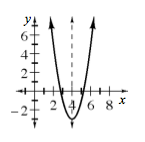### Home > A2C > Chapter 4 > Lesson 4.2.1 > Problem4-76

4-76.

Sketch a graph and draw the line of symmetry for the equation $y=2(x−4)^2−3$. What is the equation of the line of symmetry?

Use the $h$ and $k$ values in the equation to find the vertex.

Use the vertex to locate the axis of symmetry and write an equation for it.

The line of symmetry is $x=4$.